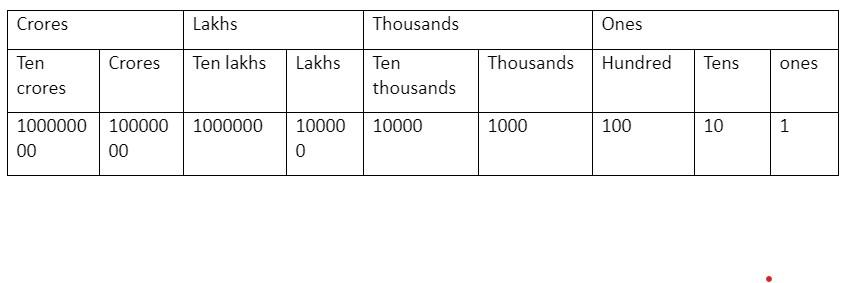Courses
Courses for Kids
Free study material
Free LIVE classes
MoreLIVE
Join Vedantu’s FREE Mastercalss

# Fill in the blank.1 lakh = _____ ten thousand.Verified
360.3k+ views
Hint: First of all, let there be ‘n’ ten thousand in 1 lakh. Then by place value system, write 1 lakh = 100000 and ten thousand = 10000 and divide them to get value of n.

Here we have to find the number of ten thousand in 1 lakh fill the blank, 1 lakh_____ ten thousand.
Before proceeding with this question, we must know what numbering system is.
Numbering system is basically the system which is used to express the numbers. This contains words and symbols to express different numbers. Similarly Indian numbering system is used in the Indian subcontinent to express numbers.
For example lakhs and crores are most commonly used terms to express large numbers in the system.
We differentiate different numbers and their digits by stating their ‘place value’. ‘Place value’ is defined as the numerical value of a digit on the basis of its position in a number.
For example if we take a number 445, the digit 4 is used twice in this number. Both of them have a different value. We differentiate them by stating their place value. The place value of leftmost 4 is hundred while the one in centre is Tens and place value of 5 is units/ones. Hence, we call 445 as four hundred and forty five and we can also write, 445 = 4x100 + 4x10 + 5x1.

Therefore, we get place value chart as,For example in number, 23,45,67,819
9 is at one place.
1 is at tens place.
8 is a hundred place.
7 is in a thousand place.
6 is at ten thousand place.
5 is at lakhs place.
4 is at ten lakh place.
3 is at crores place.
2 is at ten crore place.
In question, we have to find the number of ten thousand in 1 lakh.
From place value chart, we can write 1 lakh as,
1 lakh = 100000
Also from same chart, we can write thousand as,
Ten thousand = 10000
Let us suppose that there are ‘n’ ten thousand in 1 lakh, we get,
1 lakh$=\underline{n}.ten\ thousand$
We can also write it as
100000 = n.(10000)
By dividing 10000 on both sides, we get,
$\Rightarrow \dfrac{100000}{10000}=n$
Or n = 10
Hence, there are 10 ten thousand in 1 lakh.
So, we can write 1 lakh = 10 ten thousand.

Note: Students often make mistakes while writing the zeros at the end of the number, so this mistake must be taken care of. Also students must note the above place value system is according to Indian numbering system. In the international numbering system, we use terms like millions, and billions in place of lakhs and crores and their place value change accordingly. For example,
1 million = 10 lakhs
and 1 billion = 100 crores and so on.
Last updated date: 18th Sep 2023
Total views: 360.3k
Views today: 3.60k